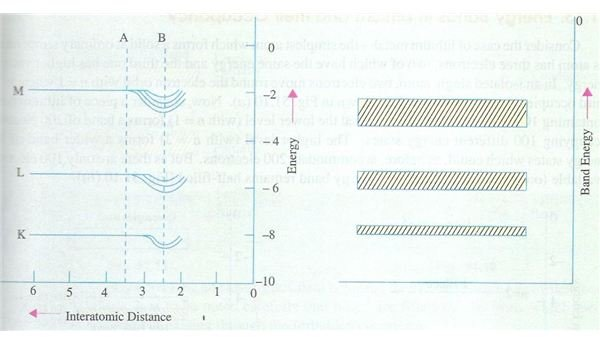# What is energy band: Learn how these bands are formed

## Introduction

In our article related to Bohr’s atomic structure theory, we learnt that electrons form discrete energy levels which were described by the mathematical formula. Yet this theory is fine when a single atom is considered in isolation, but the in real world atoms are closed packed and this produces some deviations from the theory. Now we will learn about energy bands as follows.

## What is energy band?

As per the Bohr’s theory, the electrons cannot occupy arbitrary positions in the atomic space surrounding the nucleus but only exist in predefined discrete levels. Now consider what happens when two or more atoms come closer and each has their discrete energy levels. Since the electromagnetic forces will come into play as the distance between the atoms decreases, the energy levels will get affected by this mutual interaction.

As the inter atomic distance goes on decreasing, the influence of these forces increases and the net result is that instead of discrete energy levels, we now have a narrow range of energy band corresponding to each energy level, and these bands are known as energy bands.If you find this concept a bit confusing, just refer to the diagram shown here which clearly shows the energy levels labeled as K, L and M on the left hand side of the diagram, whilst the right hand side shows the energy bands corresponding to these energy levels; the inter atomic distance being shows on the X-axis on the left hand side. You will also notice that the M level band is broader than the inner band and so on. This is obviously due to the fact that as the atoms start to come closer, the valence level gets affected first due to mutual repulsion between electrons of both atoms, while the inner levels are affected to a relatively lesser degree. Hence the variation in the outermost levels is more than the inner levels, hence the explanation for this observation. In case you are not fully familiar with the energy levels K, L, M etc just refer to the article on energy shells.

## Continuous or discrete

Of course it must also be noted that though the energy band has been shown as a continuous band and it can be considered so for broad purposes, in actual practice it is not so. The various energy bands consist of discrete energy levels which are closely coupled and are only slightly different from each other. To get an idea of how closely these discrete bands within a single band are located, let us take a look at a typical example of a crystal which contains 2*1019 levels within the s-band. This means that the average spacing between the energy levels of this band would be of the order of 10-19 eV.

In case you are not familiar with eV, this refers to electron volts which is a unit of energy and also used as unit of mass etc. We will study about this in detail in a separate article.

Energy band in gases

You must also be aware that the atoms of a gas are much loosely bound than those of solids and liquids. Since the above mentioned effect occurs mainly due to close coupling of atoms, it would be interesting to note that under normal conditions of pressure and temperature, there is no band formation in the atoms of the gases and the energy levels are only discretely defined, as per the theoretical analysis of Neils Bohr.

Having learnt about the energy bands in general, we will proceed to learn about valence and conduction bands which would help us to know more about the basic principles behind digital electronics.

## Image Credits

Theraja, B.L. & Theraja, A.K. (2006) Electrical Technology Vol IV. New Delhi: S.Chand and Company Publications